# LR Sequencing: Cookies

You are here: Home  CAT Questionbank   LR Sequencing Puzzles  Cookies Q.5

## LR Sequencing Puzzles: Cookies and Biscuits

Five students, P, Q, R, S and T stand in a line in some order and receive cookies and biscuits to eat. No student gets the same number of cookies or biscuits. The person first in the queue gets the least number of cookies. Number of cookies or biscuits received by each student is a natural number from 1 to 9 with each number appearing at least once.

How many cookies were distributed in all?

25 cookies were distributed in total.

## Detailed Solution

General Solution

Let us make the some inferences first, then we will build the table and work from there.

Number of cookies or biscuits received by each student is a natural number from 1 to 9 with each number appearing at least once. – Each number from 1 to 9 appears once, with one number appearing twice.

T receives 8 more cookies than biscuits – T receives 9 cookies and 1 biscuit The person who is last in the queue received 10 items in all, while P receives only half as many totally – P receives only 5 items totally. Either 1 + 4 or 2 + 3 in some order. Number of cookies Q receives is equal to the number of biscuits P receives – This is the number that appears twice. So, the number that appears twice is either 1, 2, 3 or 4. R who was in the middle of the line => R is third.

The total number of cookies is two more than the total number of biscuits distributed. => This is a very vital clue. This tells us that the total number of cookies and biscuits are both odd or both even. Importantly, the total number of cookies + total number of biscuits is an even number.

1+ 2 + 3 + … 9 = 45. So, if the total is an even number, the number that repeats should be odd. We know that the number that appears twice has to be 1, 2, 3 or 4. So, we can further narrow this to 1 or 3. Further, we know that T receives 9 cookies and 1 biscuit. So, the number that repeats is 3. So, number of cookies Q gets = number of biscuits P receives = 3. Number of cookies P receives should be 2.

The overall total number is 48. Total number of cookies = 25, total number of biscuits = 23. Let us consolidate the data we have.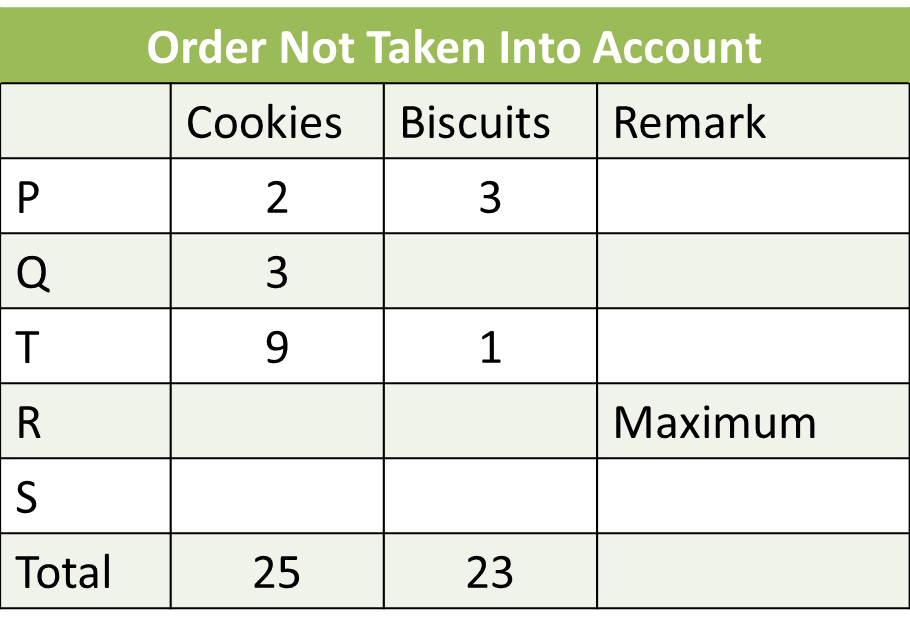The person first in the queue receives the least number of cookies. Or, P should be first in the queue. R is third. Q is before S in the queue.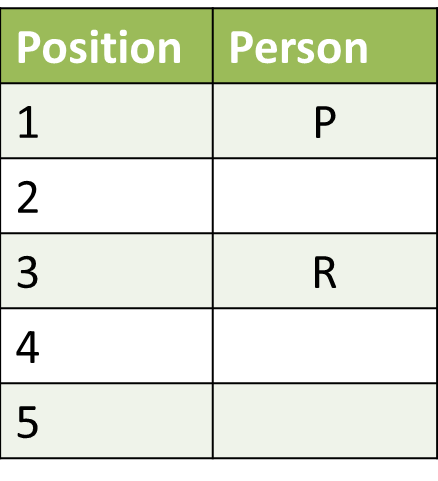Q receives one more good than S and one less than R. T receives 10 and P receives 5. So, Q, R and S together should receive 48 – 15 = 33. Q receives one more good than S and one less than R. Or, Q should receive 11, R should receive 12 and S should receive 10. Either S or T should be last in the queue.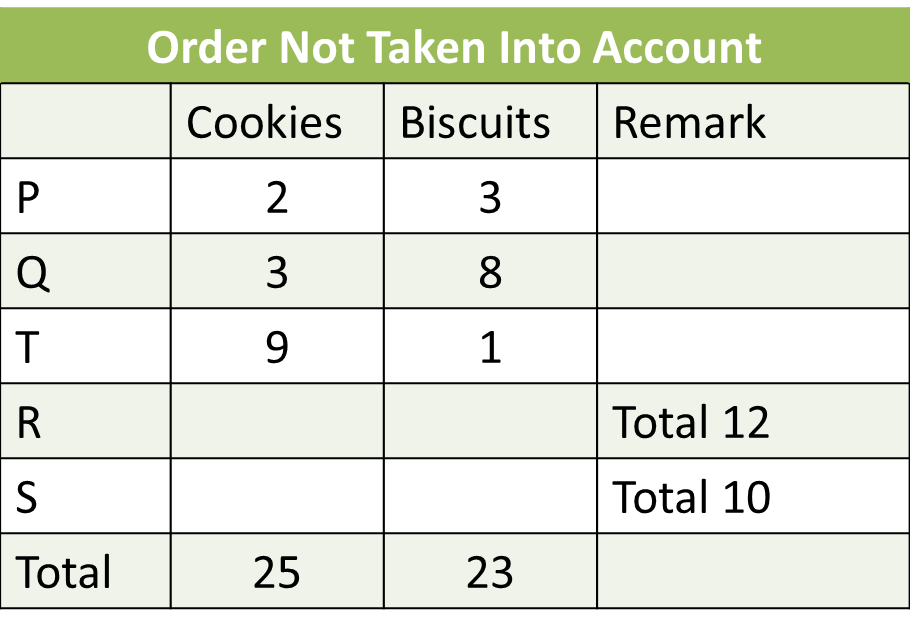Q is before S in the queue. Or Q is not last. Person second in the queue receives an odd number of biscuits and an odd number of cookies. Q receives 3 cookies and 8 biscuits, so Q is not second. So, Q has to be 4th, Q is before S, so S is last. T is second.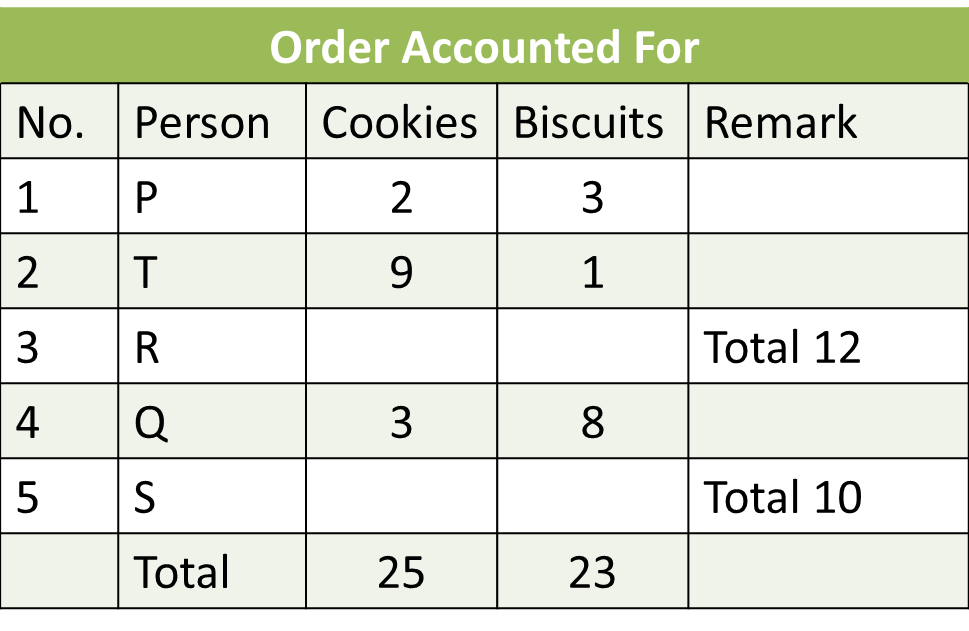The numbers missing are 4, 5, 6 and 7. R should have received 5 and 7. S should have received 4 and 6. Since the total numbers should also match, we can arrive at two possibilities overall.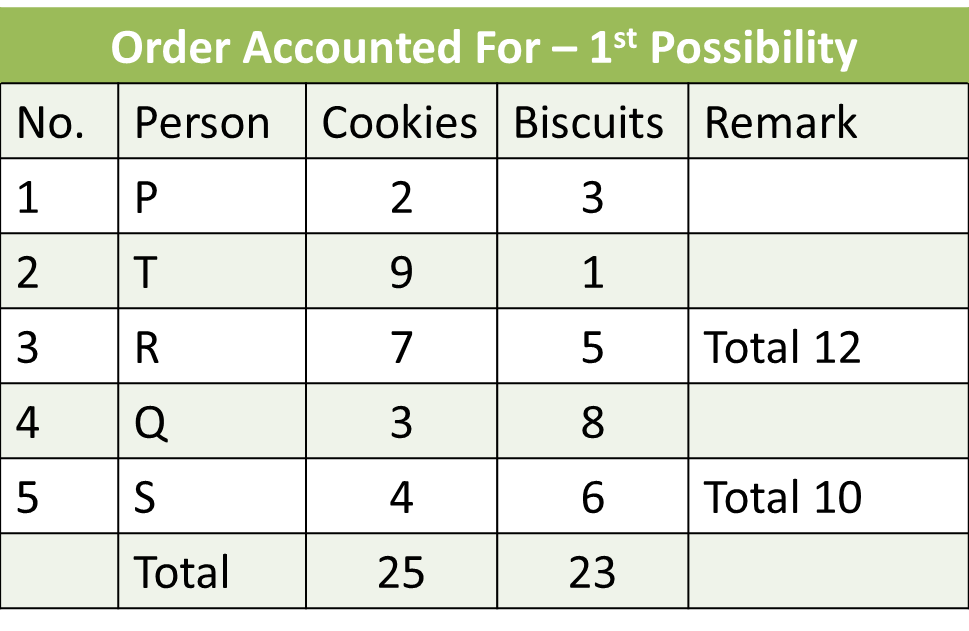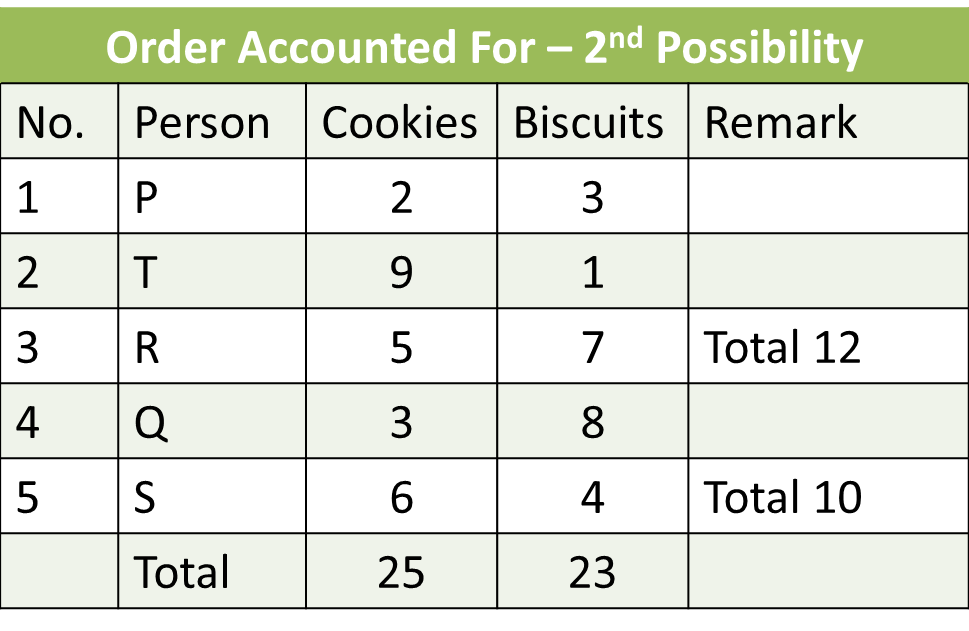Solution to Question 5

## Our Online Course, Now on Google Playstore!### Fully Functional Course on Mobile

All features of the online course, including the classes, discussion board, quizes and more, on a mobile platform.

### Cache Content for Offline Viewing

Download videos onto your mobile so you can learn on the fly, even when the network gets choppy!